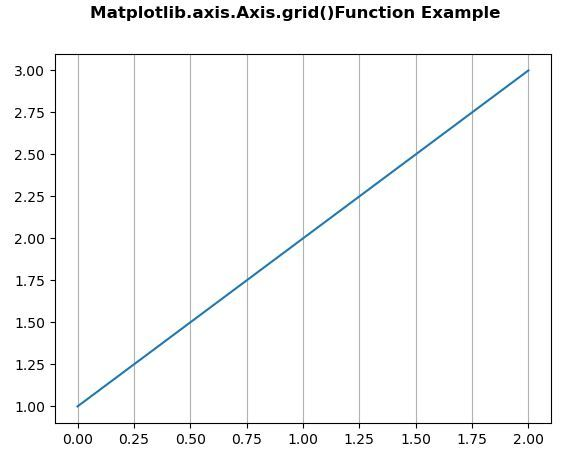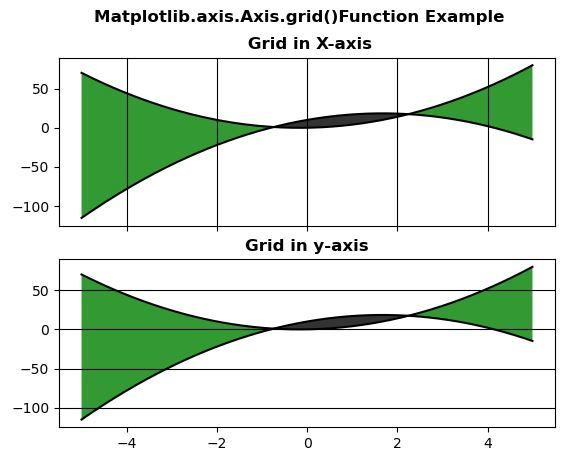Related Articles

# Matplotlib.axis.Axis.grid() function in Python

• Last Updated : 03 Jun, 2020

Matplotlib is a library in Python and it is numerical – mathematical extension for NumPy library. It is an amazing visualization library in Python for 2D plots of arrays and used for working with the broader SciPy stack.

## matplotlib.axis.Axis.grid() Function

The Axis.grid() function in axis module of matplotlib library is used to Configure the grid lines.

Syntax: Axis.grid(self, b=None, which=’major’, **kwargs)

Parameters: This method accepts the following parameters.

• b : This parameter is an optional parameter, whether to show the grid lines or not.
• which : This parameter is also an optional parameter and it is the grid lines to apply the changes on.

Return value: This method does not return any value.

Below examples illustrate the matplotlib.axis.Axis.grid() function in matplotlib.axis:
Example 1:

## Python3

 `# Implementation of matplotlib function ``from` `matplotlib.axis ``import` `Axis  ``import` `matplotlib.pyplot as plt ``import` `numpy as np ``      ` `fig, ax ``=` `plt.subplots() ``ax.plot([``1``, ``2``, ``3``]) ``ax.xaxis.grid() ``   ` `fig.suptitle("Matplotlib.axis.Axis.grid()\``Function Example", fontsize ``=` `12``, fontweight ``=``'bold'``)`` ` `plt.show()`

Output:Example 2:

## Python3

 `# Implementation of matplotlib function ``from` `matplotlib.axis ``import` `Axis  ``import` `numpy as np ``import` `matplotlib.pyplot as plt ``     ` `x ``=` `np.arange(``-``5``, ``5``, ``0.01``) ``y1 ``=` `-``3` `*` `x``*``x ``+` `10` `*` `x ``+` `10``y2 ``=` `3` `*` `x``*``x ``+` `x ``     ` `fig, [ax, ax1] ``=` `plt.subplots(``2``, ``1``,  ``                              ``sharex ``=` `True``) ``   ` `ax.plot(x, y1, x, y2, color ``=``'black'``) ``ax.fill_between(x, y1, y2, where ``=` `y2 >y1, ``                ``facecolor ``=``'green'``, ``                ``alpha ``=` `0.8``) ``   ` `ax.fill_between(x, y1, y2, where ``=` `y2 <``=` `y1,  ``                ``facecolor ``=``'black'``,  ``                ``alpha ``=` `0.8``) ``   ` `ax.xaxis.grid(``True``, color ``=``"black"``) ``ax.set_title(``'\n Grid in X-axis'``, ``             ``fontsize ``=` `12``, fontweight ``=``'bold'``) ``   ` `ax1.plot(x, y1, x, y2, color ``=``'black'``) ``ax1.fill_between(x, y1, y2, where ``=` `y2 >y1,  ``                 ``facecolor ``=``'green'``, ``                 ``alpha ``=` `0.8``) ``   ` `ax1.fill_between(x, y1, y2, where ``=` `y2 <``=` `y1, ``                 ``facecolor ``=``'black'``,  ``                 ``alpha ``=` `0.8``) ``   ` `ax1.yaxis.grid(``True``, color ``=``"black"``)``ax1.set_title(``'Grid in y-axis'``, ``             ``fontsize ``=` `12``, fontweight ``=``'bold'``) ``   ` `fig.suptitle("Matplotlib.axis.Axis.grid()\``Function Example", fontsize ``=` `12``, fontweight ``=``'bold'``) `` ` `plt.show()`

Output:Attention geek! Strengthen your foundations with the Python Programming Foundation Course and learn the basics.

To begin with, your interview preparations Enhance your Data Structures concepts with the Python DS Course. And to begin with your Machine Learning Journey, join the Machine Learning – Basic Level Course

My Personal Notes arrow_drop_up